# MOSFET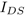-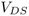characteristics

• Linear region:• Saturation: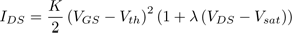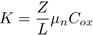S2

cm2V-1s-1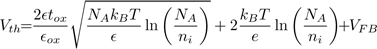· 10
· 10close

## MOSFET-characteristics

Just skip this information and continue with the plot anyway, this help may be shown by clicking the    -icon.

This application plots the-characteristics of a n-channel MOSFET according to the input data characterizing the transistor and its functional state.

### How to use this application

On the right side of the screen the desired settings may be inputted. On changing a value, the plot on the left side automatically changes by recalculating the transistor equations described below. More than one curve parametrized by the gate-source voltage may be plotted at once. To access this help click the    -icon in the top right corner. It may be closed by clicking here or by using the close label in the top right corner of this overlay.

### MOSFET drain currentThe formula for the drain currentis derived with the gradual channel approximation. This model assumes a voltage drop across the channel caused by the outer drain-source voltagewhich shrinks the conducting channel limiting the current. This approximation leads to the following formula:withcontaining the transistor's geometry and material properties.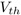is the threshold voltage of the transistor describing the minimum gate voltage for strong inversion and thus starting to conduct:Note that in the formula above the intrinsic carrier concentration is temperature dependent as well. This application correctly consideres this fact, so no action has to be taken by the user when changing temperature.

The formula forabove describes an upside down parabola. Experiments show that the drain current does not decrease as the formula suggests whengets bigger and bigger. Ifgets bigger than the so called saturation voltage (which is the drain-source voltage at the maximum drain current), the transistor is said to be in saturation. Otherwise it's said to be in linear operation. In saturation, the current through the transistor can not be increased by an increase in drain source voltage. It basically acts as a voltage controlled current source. The current drive of the transistor in the saturation region can be calculated with the following equation:Note the extra term withinside. This quantity is called the channel length modulation coefficient. Experiments show that the drain current slightly increases when increasing the drain-source voltage in saturation. So a MOSFET is not an ideal current source, as the current is dependent on the voltage applied. To regard this fact in the formula, this coefficient was introduced. When being zero, the transistor acts like an ideal current source. This value may only be determined experimentially, typical values lie between 0.0001V-1 and 0.1V-1.

### Parameters

•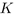K
If selected, the value of.
Z
The width of the transistor (only applies ifis calculated).
L
The gate length of the transistor (only applies ifis calculated).
μn
The electron mobility of the transistor substrate (only applies ifis calculated).
Cox
The specific capacitance of the gate oxide (only applies ifis calculated).
•: threshold voltage
Vth
If selected, the value of.
tox
The width of the gate oxide (only applies ifis calculated).
εox
The relative permettivity of the gate oxide (only applies ifis calculated).
NA
The acceptor doping concentration (only applies ifis calculated).
ni
The intrinsic carrier concentration of the substrate at 300K (only applies ifis calculated).
T
The temperature (only applies ifis calculated).
VFB
The flat band voltage of the transistor (only applies ifis calculated).
•: channel length modulation
λ
The channel length modulation.
•: gate-source voltage
VGS
The gate source voltage. To plot more than one gate voltage, add another one with the ⊕-button.